You are here: Symbol Reference > Classifier Namespace
 Data Miner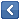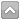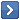Classifier Namespace

Contains classification components.

 Name Description The following table lists classes in this documentation. The following table lists functions in this documentation. The following table lists structs, records, enums in this documentation. The following table lists types in this documentation.
 Name Description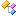Holds a list of classes for Naive Bayes classification algorithm.Classification class definition for the Naive Bayes classification algorithm.The abstract object holds a list of known classification classes.Abstract class for items of a list of clasification classes.Abstract class for encapsulation of different classification algorithms.Implementation of the K-NN (K Nearest Neighbors) classification algorithm.Holds a list of classes for the Linear Classifier classification algorithm.Classification class for the "Linear classifier" classification algorithm. Classification class for the "Linear classifier" classification algorithm.Implementation of the "Linear classifier" classifier.Implementation of the "Naive Bayes" classification algorithm.Abstract classifier class for statistical classifiers.
 Name Description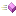Compute average of the a array.Compute standard deviation.
 Name Description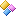Record type used to store parameteres learned for discrete attributes.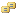Distance model used by the K-NN algorithm.Record type used by K-NN (K Nearest Neighbors) classification algorithm to store the entire learn database in memory.Record type used to store parameteres learned for continuous attributes.Used by the TClassifier to estimate the quality of the attributes.Record type used by K-NN (K Nearest Neighbors) classification algorithm to store the information about the range of real valued attributes.Record type used by K-NN (K Nearest Neighbors) classification algorithm to control the use of attributes.Record type used by K-NN (K Nearest Neighbors) classification algorithm to store the nearest neighbors.
 Name Description This is type Classifier.PAPointer. Pointer to the TKRecord type. This is type Classifier.TDiscreteRecordArray. This is type Classifier.TDoubleArray. This is type Classifier.TFloatRecordArray. Event type used by TClassifier. This is type Classifier.TIntegerArray. Event type used by TClassifier. Event type used to determine classification accuracy. Event type used to get bookmark position. This is type Classifier.TPushBookmark.